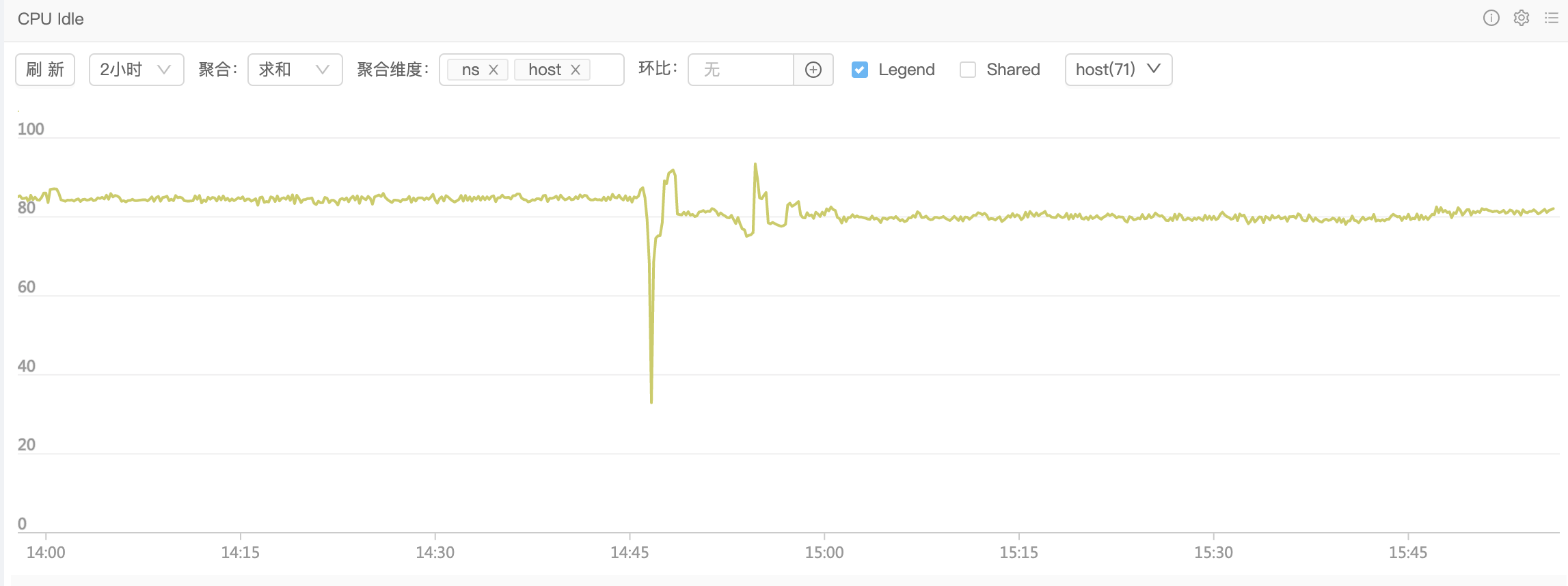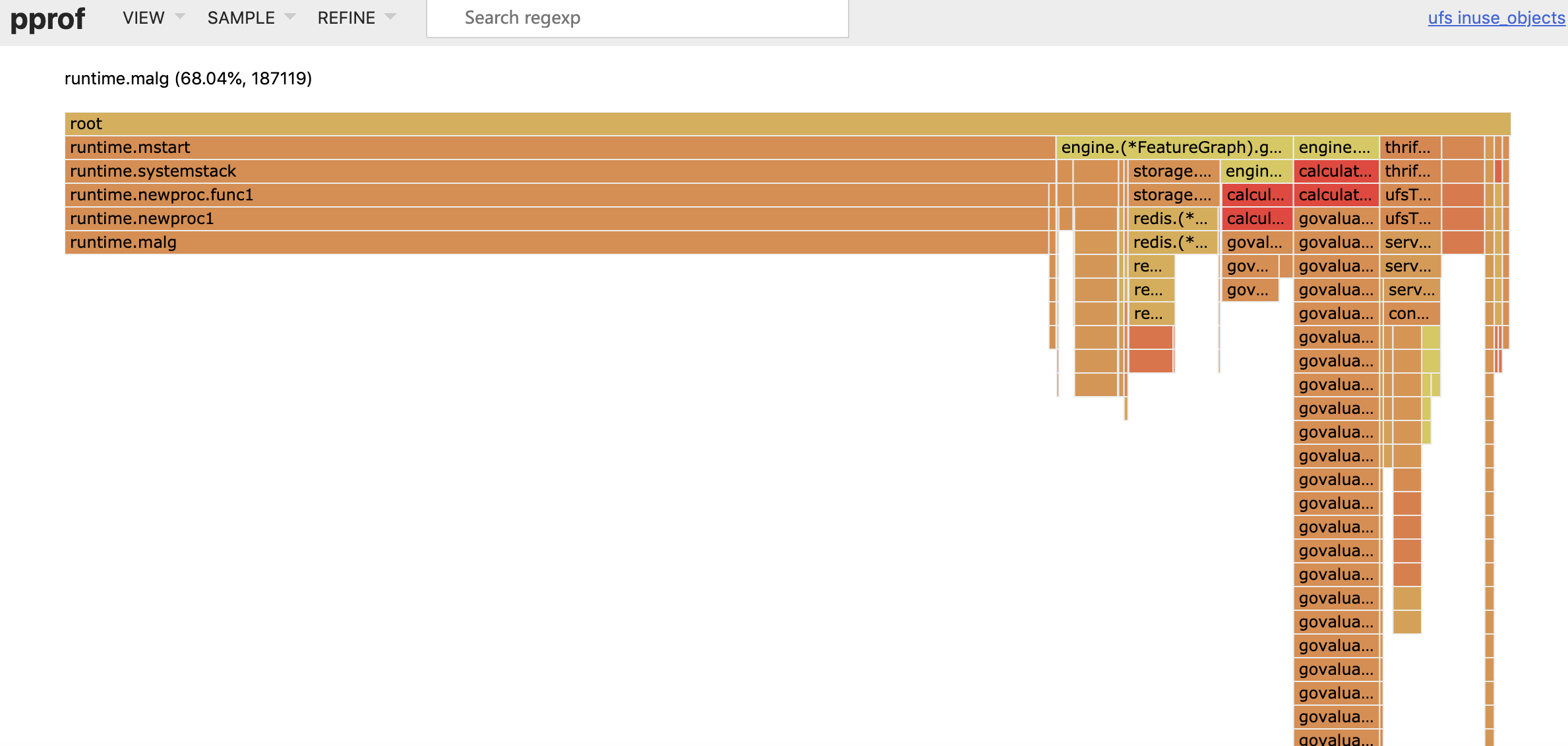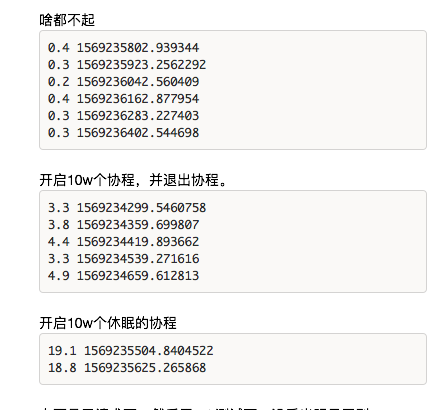``````func gfput(_p_ *p, gp *g) {
}

stksize := gp.stack.hi - gp.stack.lo

if stksize != _FixedStack {
// non-standard stack size - free it.
stackfree(gp.stack)
gp.stack.lo = 0
gp.stack.hi = 0
gp.stackguard0 = 0
}

_p_.gFree.push(gp)
_p_.gFree.n++
if _p_.gFree.n >= 64 {
lock(&sched.gFree.lock)
for _p_.gFree.n >= 32 {
_p_.gFree.n--
gp = _p_.gFree.pop()
if gp.stack.lo == 0 {
sched.gFree.noStack.push(gp)
} else {
sched.gFree.stack.push(gp)
}
sched.gFree.n++
}
unlock(&sched.gFree.lock)
}
}

func gfget(_p_ *p) *g {
retry:
if _p_.gFree.empty() && (!sched.gFree.stack.empty() || !sched.gFree.noStack.empty()) {
lock(&sched.gFree.lock)
for _p_.gFree.n < 32 {
// Prefer Gs with stacks.
gp := sched.gFree.stack.pop()
if gp == nil {
gp = sched.gFree.noStack.pop()
if gp == nil {
break
}
}
sched.gFree.n--
_p_.gFree.push(gp)
_p_.gFree.n++
}
unlock(&sched.gFree.lock)
goto retry
}
gp := _p_.gFree.pop()
if gp == nil {
return nil
}
_p_.gFree.n--
if gp.stack.lo == 0 {
systemstack(func() {
gp.stack = stackalloc(_FixedStack)
})
gp.stackguard0 = gp.stack.lo + _StackGuard
} else {
// ....
}
return gp
}
``````

``````func newproc1(fn *funcval, argp *uint8, narg int32, callergp *g, callerpc uintptr) {
_g_ := getg()

// .... 省略无关代码

_p_ := _g_.m.p.ptr()
newg := gfget(_p_)
if newg == nil {
newg = malg(_StackMin)
}
}
``````

``````var (
allgs    []*g
allglock mutex
)
``````

GC 的时候：

``````func gcResetMarkState() {
lock(&allglock)
for _, gp := range allgs {
gp.gcscandone = false  // set to true in gcphasework
gp.gcscanvalid = false // stack has not been scanned
gp.gcAssistBytes = 0
}
}
``````

``````func checkdead() {
// ....
grunning := 0
lock(&allglock)
for i := 0; i < len(allgs); i++ {
gp := allgs[i]
if isSystemGoroutine(gp, false) {
continue
}
}
}
``````

1. 下游系统超时，很多 g 都被阻塞了，挂在 gopark 上，相当于提高了系统的并发
2. 因为 gFree 没法复用，导致创建了比平时更多的 goroutine(具体有多少，就看你超时设置了多少
3. 抖动时创建的 goroutine 会进入全局 allgs 数组，该数组不会进行收缩，且每次 gc、sysmon、死锁检查期间都会进行全局扫描
4. 上述全局扫描导致我们的系统在下游系统抖动恢复之后，依然要去扫描这些抖动时创建的 g 对象，使 cpu 占用升高，idle 降低。
5. 只能重启(重启大法好

``````package main

import (
"log"
"net/http"
_ "net/http/pprof"
"time"
)

func sayhello(wr http.ResponseWriter, r *http.Request) {}

func main() {
for i := 0; i < 1000000; i++ {
go func() {
time.Sleep(time.Second * 10)
}()
}
http.HandleFunc("/", sayhello)
err := http.ListenAndServe(":9090", nil)
if err != nil {
log.Fatal("ListenAndServe:", err)
}
}
````````````import psutil
import time

p = psutil.Process(1) # 改成你自己的 pid 就行了

while 1:
v = str(p.cpu_percent())
if "0.0" != v:
print(v, time.time())
time.sleep(1)

``````